# 1、前言

HashMap 1.7源码分析：https://carsonho.blog.csdn.net/article/details/79373026

HashMap 1.8源码分析：https://blog.csdn.net/carson_ho/article/details/79373134

简单回顾一下 HashMap 的结构：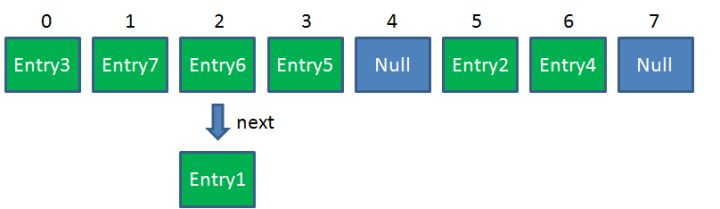在 JDK7 下，高并发时，有可能出现下面的环形链表：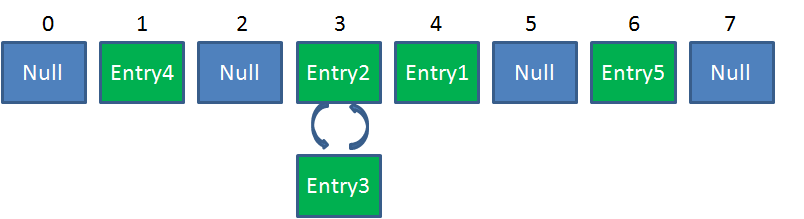要避免 HashMap 的线程安全问题，有多个解决方法，比如改用 HashTable 或者 Collections.synchronizedMap() 方法。

但是这两者都有一个问题，就是性能，无论读还是写，他们两个都会给整个集合加锁，导致同一时间的其他操作阻塞。

ConcurrentHashMap 的优势在于兼顾性能和线程安全，一个线程进行写操作时，它会锁住一小部分，其他部分的读写不受影响，其他线程访问没上锁的地方不会被阻塞。

# 2、什么是ConcurrentHashMap

`java.util.concurrent.ConcurrentHashMap`属于 JUC 包下的一个集合类，可以实现线程安全。

它由多个 Segment 组合而成。Segment 本身就相当于一个 HashMap 对象。同 HashMap 一样，Segment 包含一个 HashEntry 数组，数组中的每一个 HashEntry 既是一个键值对，也是一个链表的头节点。

单一的 Segment 结构如下：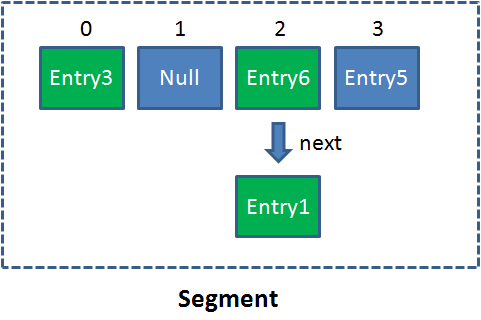像这样的 Segment 对象，在 ConcurrentHashMap 集合中有多少个呢？有 2 的 N 次方个，共同保存在一个名为 segments 的数组当中。

因此整个ConcurrentHashMap的结构如下：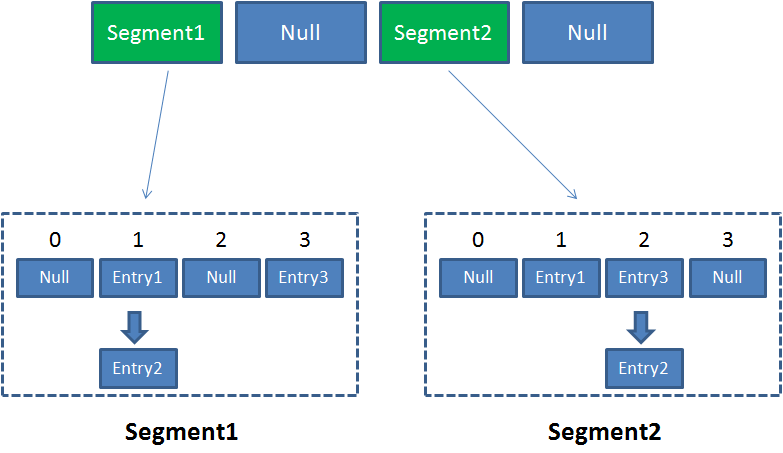可以说，ConcurrentHashMap 是一个二级哈希表。在一个总的哈希表下面，有若干个子哈希表。

它的核心属性：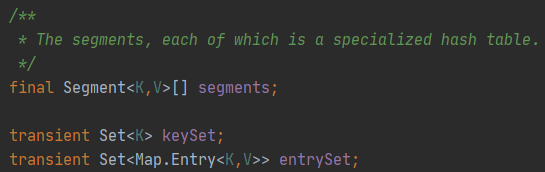``// 继承的ReentrantLock可以把Segment看作是一把锁而且还是一个容器static final class Segment<K,V> extends ReentrantLock implements Serializable {    private static final long serialVersionUID = 2249069246763182397L;    // 和 HashMap 中的 HashEntry 作用一样，真正存放数据的桶    transient volatile HashEntry<K,V>[] table;    transient int count;    transient int modCount;    transient int threshold;    final float loadFactor;    // ...}``

`HashEntry`也是一个内部类，主要组成如下：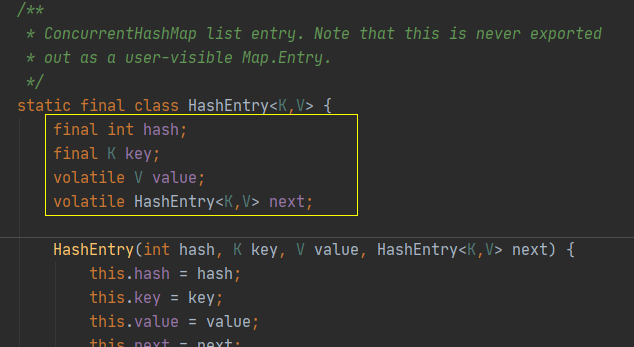和 HashMap 的 Entry 基本一样，唯一的区别就是其中的核心数据如 value ，以及链表都是 volatile 修饰的，保证了获取时的可见性。

这里不介绍构造函数。

# 3、Put 操作

``public V put(K key, V value) {    Segment<K,V> s;    if (value == null)        throw new NullPointerException();    // 计算key的hash值    int hash = hash(key);    // 根据计算的hash值计算segment数组的位置index    int j = (hash >>> segmentShift) & segmentMask;    if ((s = (Segment<K,V>)UNSAFE.getObject          // nonvolatile; recheck         (segments, (j << SSHIFT) + SBASE)) == null) //  in ensureSegment        // 获取一个segment对象        s = ensureSegment(j);    //最终调用segment的put方法进行数据存储    return s.put(key, hash, value, false);}``

``final V put(K key, int hash, V value, boolean onlyIfAbsent) {    // 加锁    HashEntry<K,V> node = tryLock() ? null :        scanAndLockForPut(key, hash, value);    V oldValue;    try {        // 内部维持的一个数组 HashEntry整体添加逻辑和HashMap差距不大        HashEntry<K,V>[] tab = table;        int index = (tab.length - 1) & hash;        HashEntry<K,V> first = entryAt(tab, index);        for (HashEntry<K,V> e = first;;) {            if (e != null) {                K k;                if ((k = e.key) == key ||                    (e.hash == hash && key.equals(k))) {                    oldValue = e.value;                    if (!onlyIfAbsent) {                        e.value = value;                        ++modCount;                    }                    break;                }                e = e.next;            }            else {                if (node != null)                    node.setNext(first);                else                    node = new HashEntry<K,V>(hash, key, value, first);                int c = count + 1;                if (c > threshold && tab.length < MAXIMUM_CAPACITY)                    rehash(node);                else                    setEntryAt(tab, index, node);                ++modCount;                count = c;                oldValue = null;                break;            }        }    } finally {        unlock(); // 释放锁    }    return oldValue;}``

虽然 HashEntry 中的 value 是用 volatile 关键词修饰的，但是并不能保证并发的原子性，所以 put 操作时仍然需要加锁处理。

首先第一步的时候会尝试获取锁，如果获取失败肯定就有其他线程存在竞争，则利用 `scanAndLockForPut()` 自旋获取锁。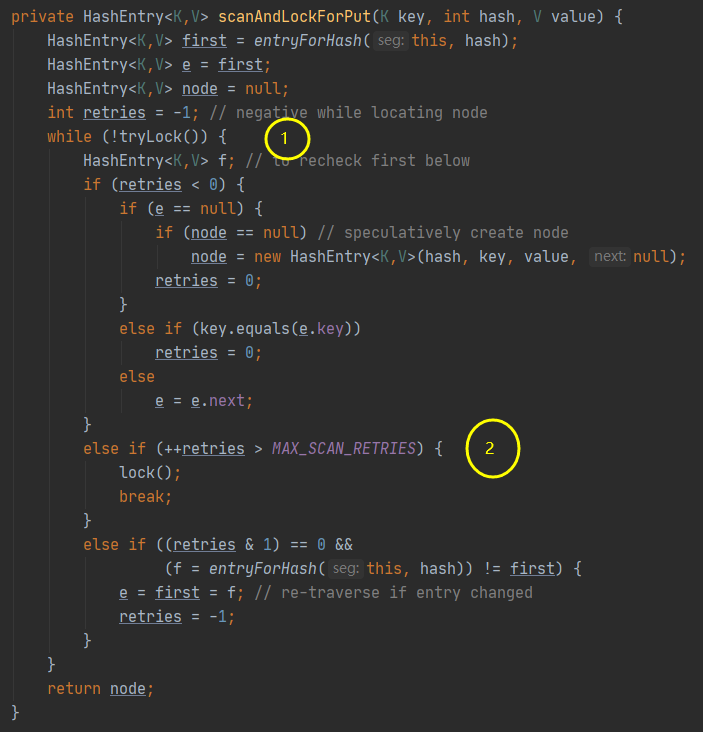1、尝试自旋获取锁。
2、如果重试的次数达到了 `MAX_SCAN_RETRIES` 则改为阻塞锁获取，保证能获取成功。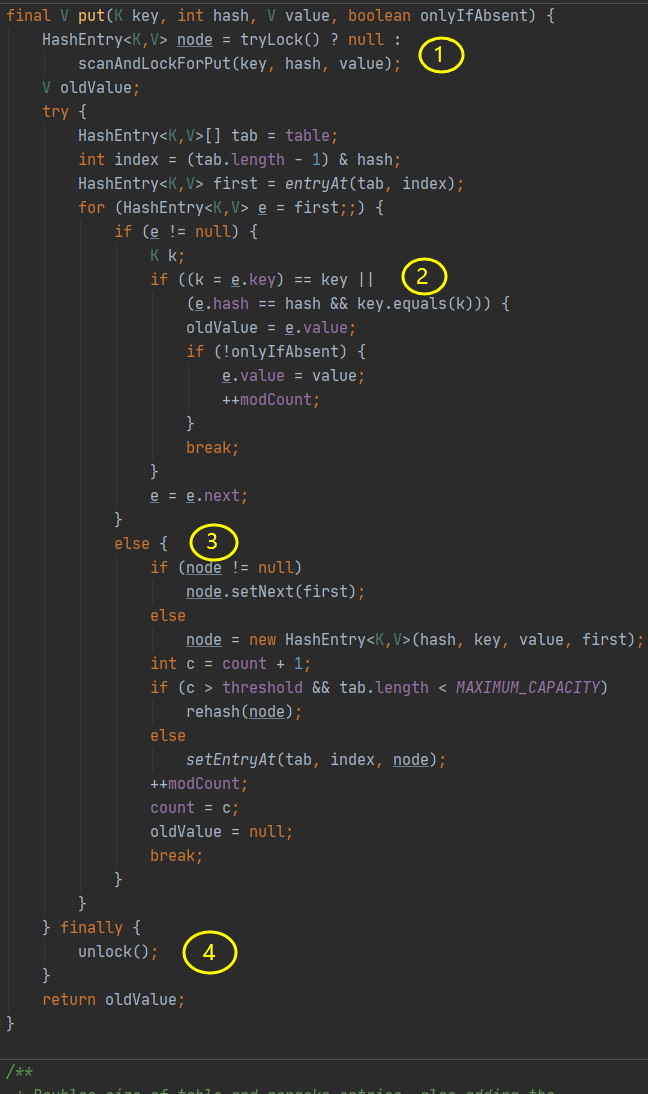1、加锁操作；
2、遍历该 HashEntry，如果不为空则判断传入的 key 和当前遍历的 key 是否相等，相等则覆盖旧的 value。
3、为空则需要新建一个 HashEntry 并加入到 Segment 中，同时会先判断是否需要扩容。
4、释放锁；

# 4、Get 操作

``public V get(Object key) {    Segment<K,V> s; // manually integrate access methods to reduce overhead    HashEntry<K,V>[] tab;    int h = hash(key);    // 计算hash值,定位到具体的segment上    long u = (((h >>> segmentShift) & segmentMask) << SSHIFT) + SBASE;    if ((s = (Segment<K,V>)UNSAFE.getObjectVolatile(segments, u)) != null &&        (tab = s.table) != null) {        for (HashEntry<K,V> e = (HashEntry<K,V>) UNSAFE.getObjectVolatile                 (tab, ((long)(((tab.length - 1) & h)) << TSHIFT) + TBASE);             e != null; e = e.next) {            K k;            if ((k = e.key) == key || (e.hash == h && key.equals(k)))                return e.value;        }    }    return null;}``

Get 操作比较简单：
1、Key 通过 Hash 之后定位到具体的 Segment；
2、再通过一次 Hash 定位到具体的元素上；
3、由于 HashEntry 中的 value 属性是用 volatile 关键词修饰的，保证了内存可见性，所以每次获取时都是最新值。

ConcurrentHashMap 的 get 方法是非常高效的，因为整个过程都不需要加锁。

# 5、高并发线程安全

Put 操作时，锁的是某个 Segment，其他线程对其他 Segment 的读写操作均不影响。因此解决了线程安全问题。

# 6、JDK8 的改进

以上介绍的是 JDK8 以前的情况，到了 JDK8 则有了一些改进。

6.1 结构改变

首先是结构上的变化，和 HashMap 一样，数组+链表改为数组+链表+红黑树。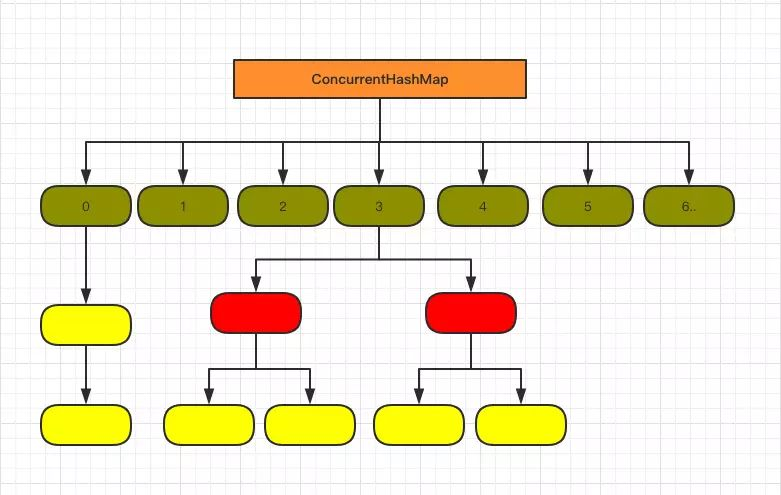6.2 HashEntry 改为 Node

``static class Node<K,V> implements Map.Entry<K,V> {    final int hash;    final K key;    volatile V val;    volatile Node<K,V> next;    ......}``

和 JDK7 的 HasEntry 作用相同，对 val 和 next 都用了 volatile 关键字，保证了可见性。

6.3 Put 操作的变化

``public V put(K key, V value) {    return putVal(key, value, false);}/** Implementation for put and putIfAbsent */final V putVal(K key, V value, boolean onlyIfAbsent) {    if (key == null || value == null) throw new NullPointerException();    // 计算hash值    int hash = spread(key.hashCode());    int binCount = 0;    for (Node<K,V>[] tab = table;;) {        Node<K,V> f; int n, i, fh;        // 数组未初始化调用#initTable进行初始化        if (tab == null || (n = tab.length) == 0)            tab = initTable();        // 要插入的元素对应位置未null,则直接CAS赋值        else if ((f = tabAt(tab, i = (n - 1) & hash)) == null) {            if (casTabAt(tab, i, null,                         new Node<K,V>(hash, key, value, null)))                break;                   // no lock when adding to empty bin        }        else if ((fh = f.hash) == MOVED)            tab = helpTransfer(tab, f);        else { // 如果有冲突            V oldVal = null;            // 加锁key对应的数组位置(其实是为所在的Node加锁)            synchronized (f) {                if (tabAt(tab, i) == f) {                    if (fh >= 0) {                        binCount = 1;                        for (Node<K,V> e = f;; ++binCount) {                            K ek;                            if (e.hash == hash &&                                ((ek = e.key) == key ||                                 (ek != null && key.equals(ek)))) {                                oldVal = e.val;                                if (!onlyIfAbsent)                                    e.val = value;                                break;                            }                            Node<K,V> pred = e;                            if ((e = e.next) == null) {                                pred.next = new Node<K,V>(hash, key,                                                          value, null);                                break;                            }                        }                    }                    else if (f instanceof TreeBin) {                        Node<K,V> p;                        binCount = 2;                        if ((p = ((TreeBin<K,V>)f).putTreeVal(hash, key,                                                       value)) != null) {                            oldVal = p.val;                            if (!onlyIfAbsent)                                p.val = value;                        }                    }                }            }            if (binCount != 0) {                if (binCount >= TREEIFY_THRESHOLD)                    // 数量大于 8 则要转换为红黑树                    treeifyBin(tab, i);                if (oldVal != null)                    return oldVal;                break;            }        }    }    addCount(1L, binCount);    return null;}``

1、根据 key 计算出 hashcode，然后开始遍历 table；
2、判断是否需要初始化；
3、f 即为当前 key 定位出的 Node，如果为空表示当前位置可以写入数据，利用 CAS 尝试写入，失败则自旋保证成功。
4、如果当前位置的 hashcode == MOVED == -1,则需要进行扩容。
5、如果都不满足，则利用 synchronized 锁写入数据。
7、如果数量大于 TREEIFY_THRESHOLD 则要转换为红黑树。

6.4 Get 操作的变化

``public V get(Object key) {    Node<K,V>[] tab; Node<K,V> e, p; int n, eh; K ek;    // 计算hash值    int h = spread(key.hashCode());    if ((tab = table) != null && (n = tab.length) > 0 &&        (e = tabAt(tab, (n - 1) & h)) != null) {        // hash匹配直接返回        if ((eh = e.hash) == h) {            if ((ek = e.key) == key || (ek != null && key.equals(ek)))                return e.val;        }        // 红黑树方式        else if (eh < 0)            return (p = e.find(h, key)) != null ? p.val : null;        // 链表方式        while ((e = e.next) != null) {            if (e.hash == h &&                ((ek = e.key) == key || (ek != null && key.equals(ek))))                return e.val;        }    }    return null;}``

1、根据计算出来的 hashcode 寻址，如果就在桶上那么直接返回值。
2、如果是红黑树那就按照树的方式获取值。
3、都不满足那就按照链表的方式遍历获取值。

6.5 总结

1.8 在 1.7 的数据结构上做了大的改动，采用红黑树之后可以保证查询效率（O(logn)），甚至取消了 ReentrantLock 改为了 synchronized，这样可以看出在新版的 JDK 中对 synchronized 优化和使用时机是很到位的。

##### 热门推荐
•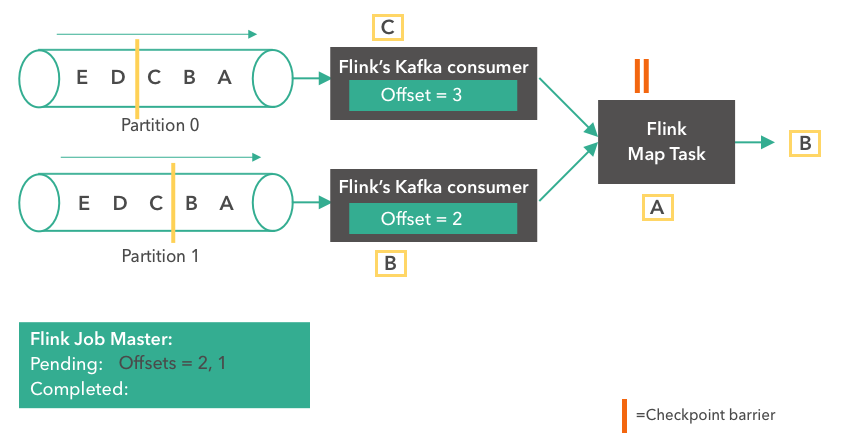浏览(2,547)
•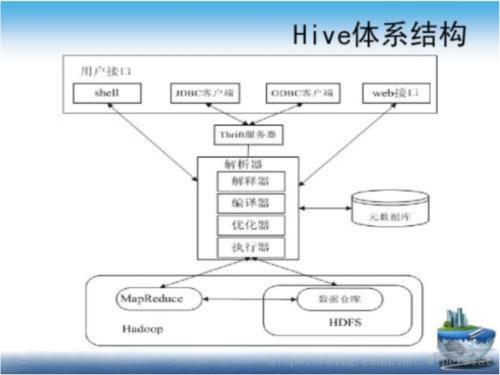浏览(1,775)
•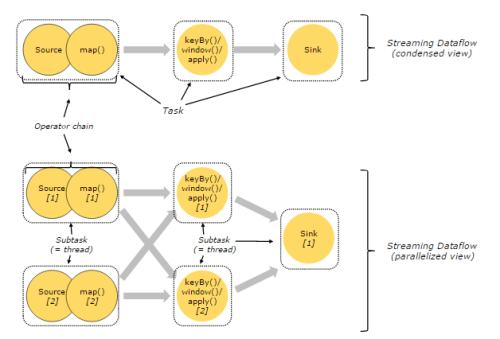浏览(1,738)
•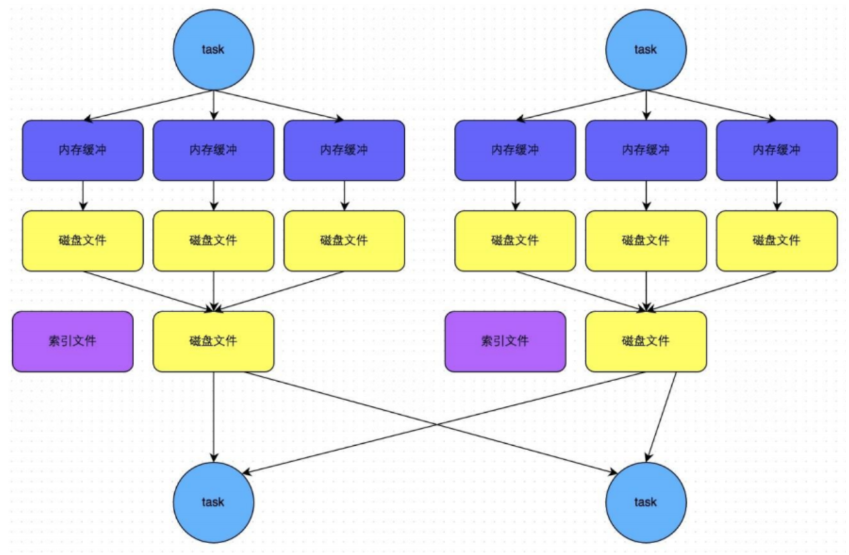浏览(1,732)
•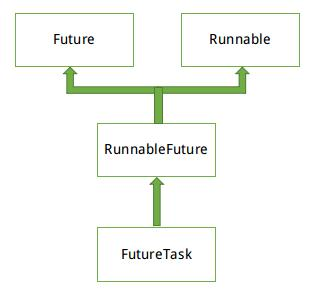浏览(1,684)
•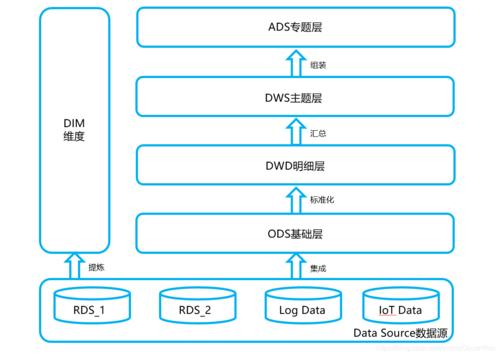浏览(1,673)
•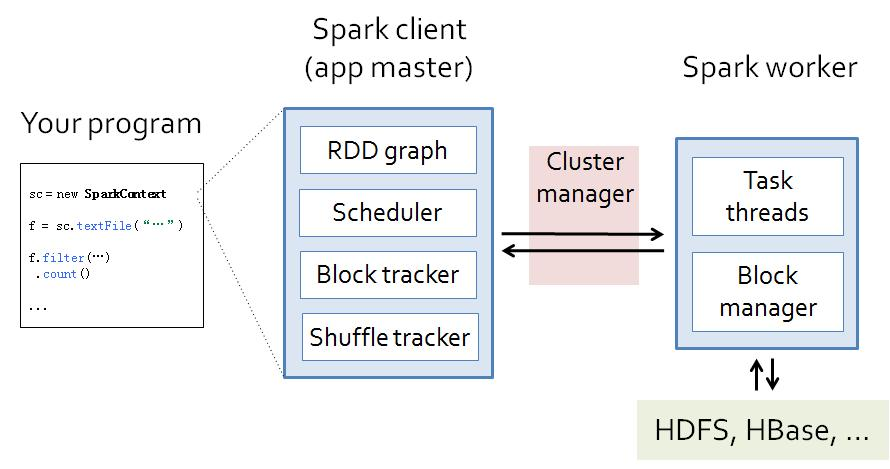浏览(1,640)
•浏览(1,613)
•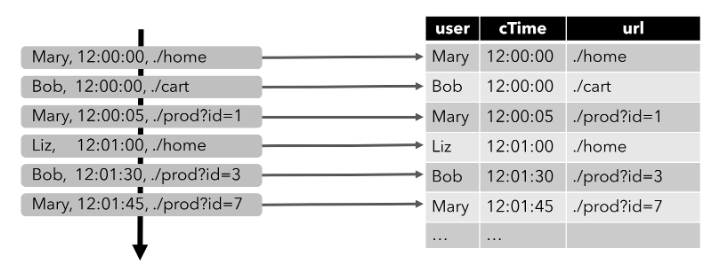浏览(1,537)
•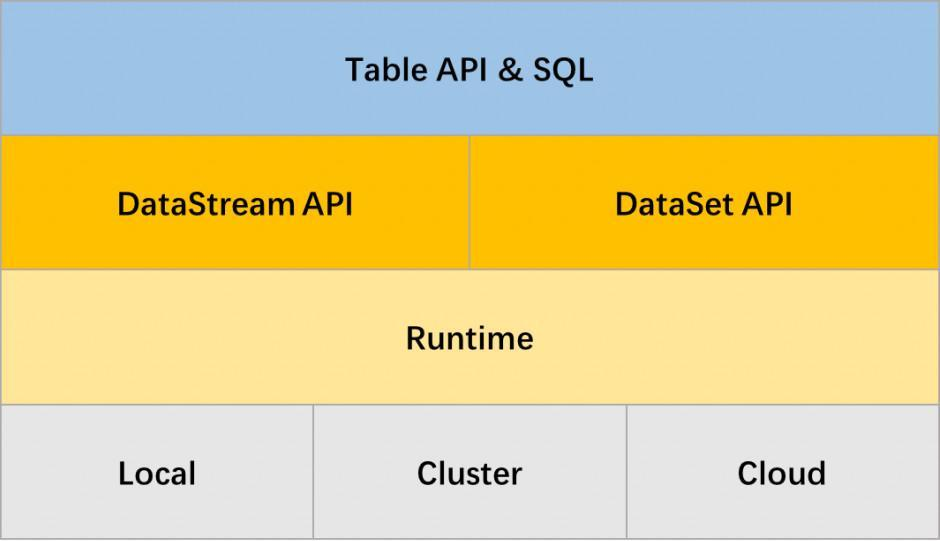浏览(1,474)